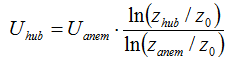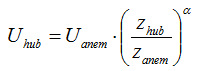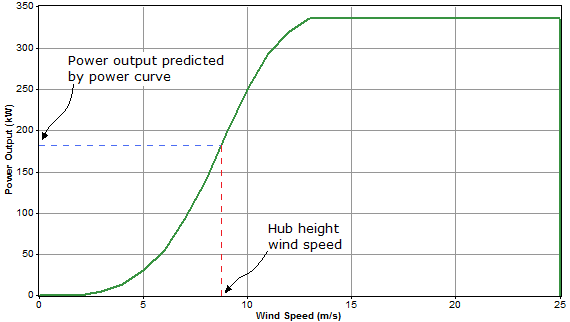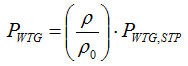# HOMER Grid 1.4

 Navigation: HOMER's Calculations How HOMER Calculates Wind Turbine Power OutputIcon

HOMER calculates the power output of the wind turbine in each time step using a three-step process. First, HOMER calculates the wind speed at the hub height of the wind turbine. Then it calculates how much power the wind turbine produces at that wind speed at standard air density. Finally, HOMER adjusts that power output value for the actual air density.

## Calculating Hub Height Wind Speed

In each time step, HOMER calculates the wind speed at the hub height of the wind turbine using the inputs you specify in the Wind Resource page and the Wind Shear entry.

If you choose to apply the logarithmic law, HOMER calculates the hub height wind speed using the following equation:where: Uhub = the wind speed at the hub height of the wind turbine [m/s] Uanem = the wind speed at anemometer height [m/s] zhub = the hub height of the wind turbine [m] zanem = the anemometer height [m] z0 = the surface roughness length [m] ln(..) = the natural logarithm

If you choose to apply the power law, HOMER calculates the hub height wind speed using the following equation:where: Uhub = the wind speed at the hub height of the wind turbine [m/s] Uanem = the wind speed at anemometer height [m/s] zhub = the hub height of the wind turbine [m] zanem = the anemometer height [m] α = the power law exponent

## Calculating Turbine Power Output At Standard Air Density

After HOMER determines the hub height wind speed, it refers to the wind turbine's power curve to calculate the expected power output from the wind turbine at that wind speed under standard conditions of temperature and pressure. In the diagram below, the red dotted line indicates the hub-height wind speed, and the blue dotted line indicates the wind turbine power output that the power curve predicts for that wind speed.

If the wind speed at the turbine hub height is not within the range defined in the power curve, the turbine produces no power, following the assumption that wind turbines produce no power at wind speeds below the minimum cutoff or above the maximum cut-out wind speeds.## Applying Density Correction

Power curves typically specify wind turbine performance under conditions of standard temperature and pressure (STP). To adjust to actual conditions, HOMER multiplies the power value predicted by the power curve by the air density ratio, according to following equation:where: PWTG = the wind turbine power output [kW] PWTG,STP = the wind turbine power output at standard temperature and pressure [kW] ρ = the actual air density [kg/m3] ρ0 = the air density at standard temperature and pressure (1.225 kg/m3)